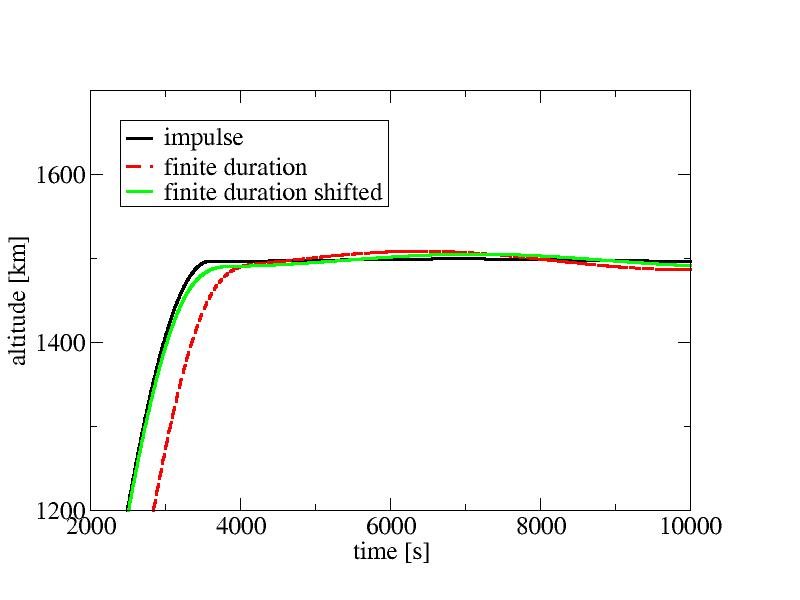# The Hohmann transfer

The Hohmann transfer is one of the basic building blocks of orbital maneuvering - it is a transfer orbit from one circular orbit to another at a different radius. The general idea is fairly simple - the spacecraft is first brought onto an elliptical orbit with an apsis just touching the target orbit, and once the apsis is reached, the spacecraft is brought to the orbital speed required for a circular orbit at that altitude. A Hohmann transfer hence requires a sequence of two burns, separated by a coasting period.

This might be a good idea to clarify terminology: In all of the following, we use the name prograde to denote a burn with the thrust vector being parallel to the velocity vector of the spacecraft, the word radial for a direction that is in the current orbital plane but perpendicular to the prograde direction and normal for the direction that completes a right-handed orthonormal system and is perpendicular to both (i.e. points directly out of the orbital plane).

For a circular orbit, a prograde burn is along the horizontal direction and a radial burn is along an imaginary line from center of the gravitating mass to spacecraft, but for eccentric orbits this relation is not exactly fulfilled.

## Programming burns

We'd like to plot a classic picture of circular orbits for once, so take the config file from the first tutorial (the 300 km orbit with 52 deg inclination) and change the plot output to

 plot2d file hohmann01.dat x x y y

while setting max_time to 6000 seconds or so. Note that this actually plots the projection of the orbit into the equatorial plane, not in the orbital plane - but this is just one overall scaling factor away.

Now we need to add the burns in. The scheme we're going to use is called PEG-7 (powered explicit guidance) and it specifies directly prograde, radial and normal velocity targets for the burn.

In spherical gravity, the Hohmann transfer requires only prograde velocity changes. Say we want to transfer from 300 km altitude to 1500 km altitude above Earth - there's simple analytical expressions (see the Wiki page linked above) which tell us the two velocities needed if we plug in these radii, the Earth radius and the gravitational constant. The first burn we can do whevever we like and the required prograde velocity to be added comes out as 312.612 s. Now, add the burn into the input section of the config file and do a plot:

We should do the second burn right at the apsis - how to find it? Easy, there is also an analytical expression for the coast time between first and second burn - so again we plug in the numbers, add the 500 seconds of the first burn, solve the delta v for the second burn and add it to the config file:

All three plots together give the classic picture of a Hohmann transfer.A Hohmann transfer from a 300 km to a 1500 km orbit.

You can also list orbital elements in the final state to see how well we're doing - the result ought to have the right altitude above ground of 1500 km and a low eccentricity. Also, plot altitude above ground vs. time and see a near-perfect insertion to a circular orbit.

## Finite burn duration

In the scenario above, we've instructed the software to apply the burns instantaneously - the velocity change happens from one timestep to the next. this is known as the impulse approximation. But it's is not how real spacecraft operate - they usually have to apply rather limited thrust. To simulate the effect of this, add

 burn_model constant

to the config section - this gives the spacecraft the acceleration of a Space Shuttle with a two engine OMS burn. Now. redo the plot of altitude vs. time. Unfortunately the precise insertion is gone - we still reach roughly the desired altitude, but with some eccentricity - and the climb happens much later.

We can mitigate the situation somewhat. Part of the issue is that applying all delta v at some time and starting an extended burn at the same time does not give the same average time of the burn. So, we might want to shift the ignition time of the extended burn forward by half the burn duration. How to find out? Look through the on-screen output, each burn reports the burn duration. Do the math, shift the ignition times forward by half the duration and plot again:Hohmann insertion for impulse approximation and finite burn duration.

Well - it clearly got better, but it's still far from a perfect insertion. How do we get the burn parameters to make this a perfect fit even for a finite-duration burn? Well, this is what a numerical targeting tool is for.

Continue with PEG-4 targeting.

Back to main index     Back to science     Back to LEO targeting

Created by Thorsten Renk 2017 - see the disclaimer, privacy statement and contact information.